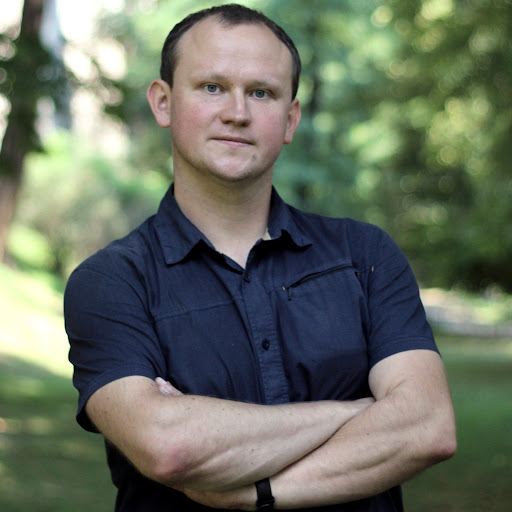12:32 AM
in The h Bar, 42 mins ago, by vzn
@Semiclassical Schroedinger vs. Navier–Stokes / de Cordoba http://www.mdpi.com/1099-4300/18/1/34
in The h Bar, 42 mins ago, by vzn
> Quantum mechanics has been argued to be a coarse-graining of some underlying deterministic theory. Here we support this view by establishing a map between certain solutions of the Schroedinger equation, and the corresponding solutions of the irrotational Navier–Stokes equation for viscous fluid flow.
in The h Bar, 42 mins ago, by vzn
> As a physical model for the fluid itself we propose the quantum probability fluid. It turns out that the (state-dependent) viscosity of this fluid is proportional to Planck’s constant, while the volume density of entropy is proportional to Boltzmann’s constant. o_O

10 hours later…10:46 AM
@CraigGidney, my physics PhD was about connection between QM and random walk ( en.wikipedia.org/wiki/Maximal_Entropy_Random_Walk ) ... but I still don't understand how Shor algorithm could work without measuring output ...
I have just looked at original Shor's paper and on page 17 he writes: "Finally, we observe the machine. It would be sufficient to observe solely the value of |c> in the first register, but for clarity we will assume that we observe both |c> and |x^a (mod n)>" ... so he agrees with you
Here is another explanation also requiring this measurement: "A measurement of the second register with the result \$k=f(s)\$ with \$s<r\$ reduces the state to (...)"
As I couldn't find it in literature, so maybe you could point calculation showing that measuring only input would also work?
I would say that not measuring the output, we should have the original ensemble on input: of all 2^n values, hence period returned by QFT would be 1.

4 hours later…3:25 PM
@Jarek was just chatting with someone locally in a QM/ QC study group who said there was a paper talking about how hard it is to measure the QFT based on smaller-and-smaller amplitudes. told him, would like to know which paper, but he didnt get back to me. wonder if there are any papers questioning the shor algorithm measurement viability/ practicality, they might be close to what youre inquiring about.

1 hour later…4:47 PM
@vzn, I am completely pessimistic regarding adiabatic QC (slide 7) - e.g. subset sum problem has "spectral gap" decreasing exponentially, while evolution time is ~ 1/"spectral gap"^2, to distinguish such local minimum we need temperature ~"spectral gap" ...
There is some hope in Shor, but there are lots of problems: decoherence requires error correction needing multiplicity of gates and so more error possibilities ... this ignored collapse of auxiliary qbits (~ N^2 of them) seems essential problem (superposition is much more complex) ... and sure reducing amplitudes seems additional problem
This low amplitude problem seems similar as in integration formulation (slide 9): if integration of prod_i cos(k_i phi) is nonzero - the problem is that nonzero means 2^-n ...

2 hours later…6:53 PM
0Adiabatic quantum computers require slow evolution to maintain global minimum: \$O(1/g^2)\$ time, where \$g\$ is spectral gap: distance between two lowest energies. The danger is that this spectral gap might decrease exponentially with problem size - this way evolution would need exponentially long ...